# Matrices Class 12 NCERT Solutions

## In this pdf file you can see answers of following Questions

### EXERCISE 3.1

Question 1. In the matrix 2 5 19 7 A 35 2 5 12 2 3 1 5 17 − = − , write:
(i) The order of the matrix,
(ii) The number of elements,
(iii) Write the elements a13, a21, a33, a24, a23.

Question 2. If a matrix has 24 elements, what are the possible orders it can have? What, if it has 13 elements?

Question 3. If a matrix has 18 elements, what are the possible orders it can have? What, if it has 5 elements?

Question 4. Construct a 2 × 2 matrix, A = [aij], whose elements are given by:
(i) ( )2 ij 2 a i j + =
(ii) ij a i j =
(iii) ( 2 )2 ij 2 a i j + =

Question 5. Construct a 3 × 4 matrix, whose elements are given by:
(i) 1| 3 | ij 2 a = − i + j
(ii) aij = 2i − j

Question 6. Find the values of x, y and z from the following equations:
(i) 4 3 5 1 5 y z x =
(ii) 2 6 2 5 5 8 x y z xy +
(iii) 9 5 7 x y z x z y z + + +

Question 7. Find the value of a, b, c and d from the equation: 2 1 5 2 3 0 13 a b a c a b c d .

Question 8. A = [aij]m × n\ is a square matrix, if
(A) m < n
(B) m > n
(C) m = n
(D) None of these

Question 9. Which of the given values of x and y make the following pair of matrices equal 3 7 5 1 2 3 x y x , 0 2 8 4
(A) 1, 7 3 x y − = =
(B) Not possible to find
(C) y = 7, 2 3 x − =
(D) 1 , 2 3 3 x y − − = =

Question 10. The number of all possible matrices of order 3 × 3 with each entry 0 or 1 is:
(A) 27
(B) 18
(C) 81
(D) 512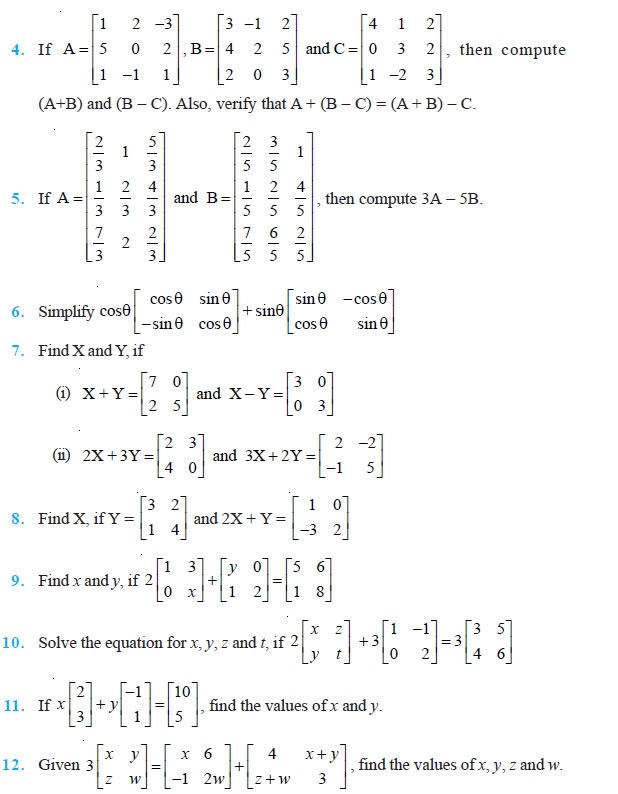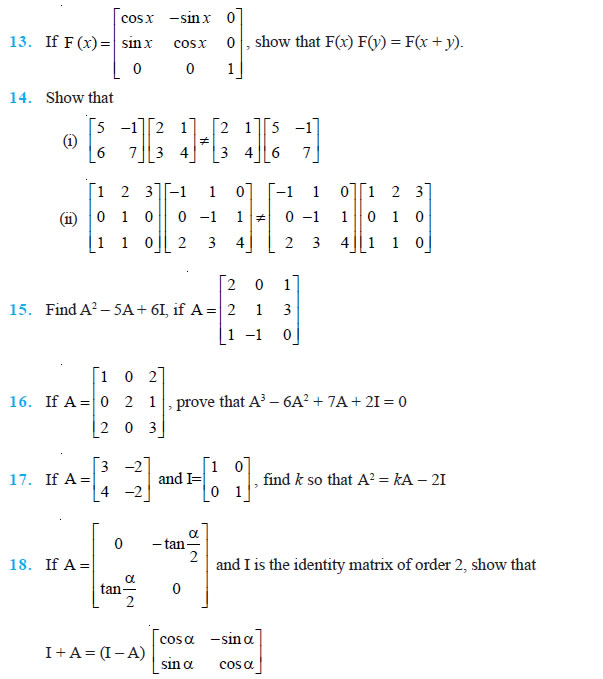Question 19. A trust fund has Rs 30,000 that must be invested in two different types of bonds. The first bond pays 5% interest per year, and the second bond pays 7% interest per year. Using matrix multiplication, determine how to divide Rs 30,000 among the two types of bonds. If the trust fund must obtain an annual total interest of:
(a) Rs 1800
(b) Rs 2000

Question 20. The bookshop of a particular school has 10 dozen chemistry books, 8 dozen physics books, 10 dozen economics books. Their selling prices are Rs 80, Rs 60 and Rs 40 each respectively . Find the total amount the bookshop will receive from selling all the books using matrix algebra. Assume X, Y, Z, W and P are matrices of order 2 × n, 3 × k, 2 × p, n × 3 and p × k, respectively. Choose the correct answer in Exercises 21 and 22.

Question 21. The restriction on n, k and p so that PY + WY will be defined are:
(A) k = 3, p = n
(B) k is arbitrary, p = 2
(C) p is arbitrary, k = 3
(D) k = 2, p = 3
22. If n = p, then the order of the matrix 7X – 5Z is:
(A) p × 2
(B) 2 × n
(C) n × 3
(D) p × n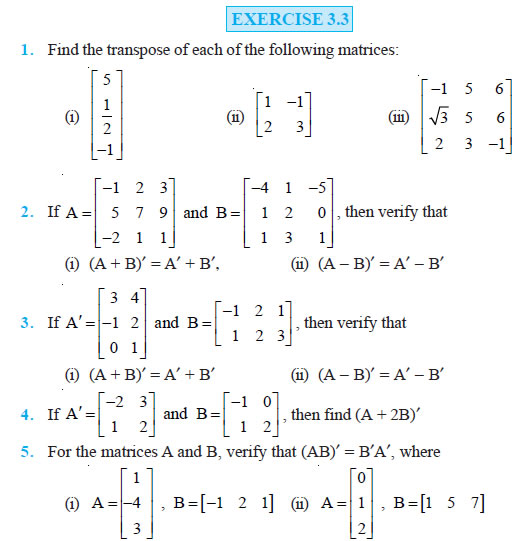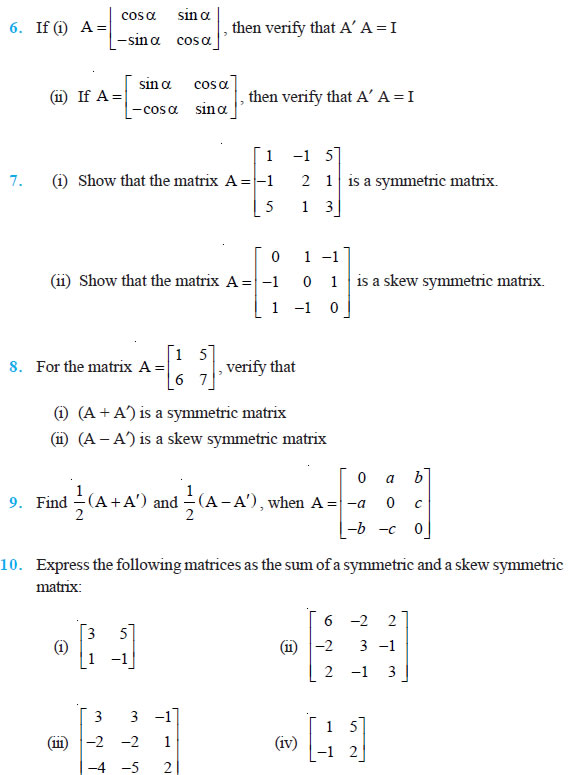Choose the correct answer in the Exercises 11 and 12.

Question 11. If A, B are symmetric matrices of same order, then AB – BA is a
(A) Skew symmetric matrix
(B) Symmetric matrix
(C) Zero matrix
(D) Identity matrix

Question 12. If cos sin A , sin cos α − α α α then A + A′ = I, if the value of α is
(A) 6 π
(B) 3 π
(C) π
(D) 3 2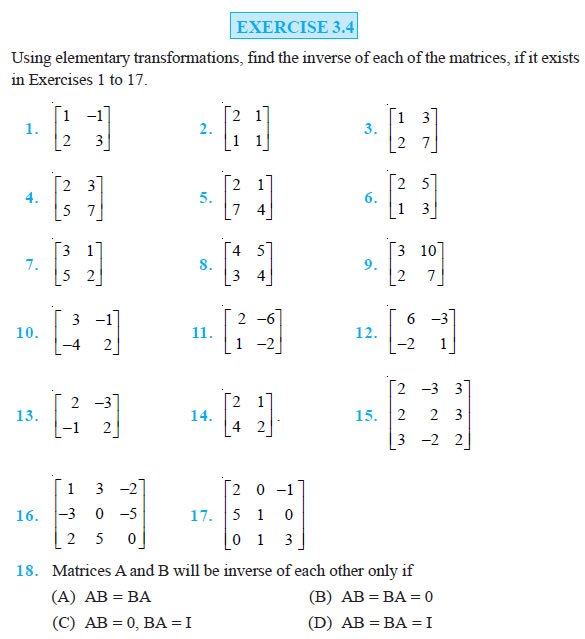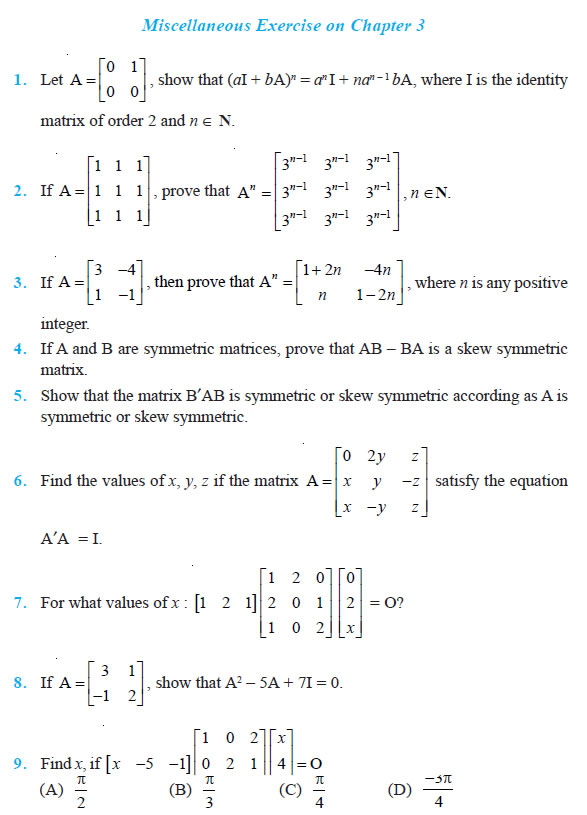Question 10. A manufacturer produces three products x, y, z which he sells in two markets. Annual sales are indicated below: Market Products I 10,000 2,000 18,000 II 6,000 20,000 8,000
(a) If unit sale prices of x, y and z are Rs 2.50, Rs 1.50 and Rs 1.00, respectively, find the total revenue in each market with the help of matrix algebra.
(b) If the unit costs of the above three commodities are Rs 2.00, Rs 1.00 and 50 paise respectively. Find the gross profit.

Question 1 1. Find the matrix X so that 1 2 3 7 8 9 X 4 5 6 2 4 6

Question 12. If A and B are square matrices of the same order such that AB = BA, then prove by induction that ABn = BnA. Further, prove that (AB)n = AnBn for all n ∈ N. Choose the correct answer in the following questions:

Question 13.
If A = α β γ α is such that A² = I, then
(A) 1 + α² + βγ = 0
(B) 1 – α² + βγ = 0
(C) 1 – α² – βγ = 0
(D) 1 + α² – βγ = 0

Question 14. If the matrix A is both symmetric and skew symmetric, then (A) A is a diagonal matrix (B) A is a zero matrix
(C) A is a square matrix
(D) None of these

Question 15. If A is square matrix such that A2 = A, then (I + A)³ – 7 A is equal to
(A) A
(B) I – A
(C) I
(D) 3A

### NCERT Books Free Pdf Download for Class 5, 6, 7, 8, 9, 10 , 11, 12 Hindi and English Medium

 Mathematics Biology Psychology Chemistry English Economics Sociology Hindi Business Studies Geography Science Political Science Statistics Physics Accountancy